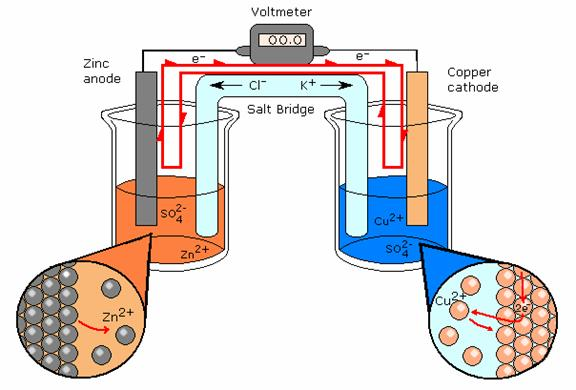Click to Chat

1800-1023-196

+91-120-4616500

CART 0

• 0

MY CART (5)

Use Coupon: CART20 and get 20% off on all online Study Material

ITEM
DETAILS
MRP
DISCOUNT
FINAL PRICE
Total Price: Rs.

There are no items in this cart.
Continue Shopping```Daniell Cell

Table of Content

Electrode Signs

Reversible and Irreversible Cells

Related ResourcesIt is designed to make use of the spontaneous redox reaction between zinc and cupric ions to produce an electric current. It consists of two half-cells. The half-cells on the left contains a zinc metal electrode dipped in ZnSO4solution.The half-cell on the right consists of copper metal electrode in a solution CuSO4. The half-cells are joined by a salt bridge that prevents the mechanical mixing of the solution.

When the zinc and copper electrodes are joined by wire, the following observations are made:

There is a flow of electric current through the external circuit.

The zinc rod loses its mass while the copper rod gains in mass.

The concentration of ZnSO4 solution increases while the concentration of copper sulphate solution decreases.

The solutions in both the compartments remain electrically neutral.During the passage if electric current through external circuit, electrons flow from the zinc electrode to the copper electrode. At the zinc electrode, the zinc metal is oxidized to zinc ions which go into the solution. The electrons released at the electrode travel through the external circuit to the copper electrode where they are used in the reduction of Cu2+ ions to metallic copper which is deposited on the electrode. Thus, the overall redox reaction is:

Zn(s) + Cu2+  →   Cu(s) + Zn2+(aq)

Thus, indirect redox reaction leads to the production of electrical energy. At the zinc rod, oxidation occurs. It is the anode of the cell and is negatively charged while at copper electrode, reduction takes, place; it is the cathode of the cell and is positively charged.

Thus, the above points can be summed up as:

Voltaic or Galvanic cell consists of two half-cells. The reactions occurring in half-cells are called half-cell reactions. The half-cell in which oxidation taking place in it is called oxidation half-cell and the reaction taking place in it is called oxidation half-cell reaction. Similarly, the half-cell occurs is called reduction half-cell and the reaction taking place in it is called reduction half-cell reaction.

The electrode where oxidation occurs is called anode and the electrode where reduction occurs is termed cathode.

Electrons flow from anode to cathode in the external circuit.

Chemical energy is converted into electrical energy.

The net reaction is the sum of two half-cell reactions. The reaction is Daniel cell can be represented as

Oxidation half reaction,

Reduction half reaction

Net reaction

Zn(s) →   Zn2+(aq) + 2e-

Cu2+(aq) + 2e- →  Cu (s)

Zn(s) + Cu2+ (aq) →  Zn2+(aq) + Cu(s)

Electrode Signs

The signs of the anode and cathode in the voltaic or galvanic cells are opposite to those in the electrolytic cells.

Electrolytic Cell

Volatic or Galvanic Cell

E.m.f. is applied to cell

E.m.f. is generated by cellElectrolytic cell

Anode  Cathode

Voltaic or Galvanic cell

Anode             Cathode

Sign

Electron flow

Half-reaction

+                   -

Out                in

Oxidation      reduction

-                         +

Out                    in

Oxidation           reduction

Reversible and Irreversible Cells

Daniell cell has the emf value 1.09 volt. If an opposing emf exactly equal to 1.09 volt is applied to the cell, the cell reaction,

Zn + Cu2+→  Cu + Zn2+

stops but if it is increased infinitesimally beyond 1.09 volt, the cell reaction is reversed.

Cu + Zn2+→  Zn + Cu2+

Such a cell is termed a reversible cell. Thus, the following are the two main conditions of reversibility:

The chemical reaction of the cell stops when an exactly equal opposing emf is applied.

The chemical reaction of the cell is reversed and the current flows in opposite direction when the opposing emf is slightly greater than that of the cell.

Any other cell which does not obey the above two conditions is termed as irreversible.

A cell consisting of zinc and copper electrodes dipped into the solution of sulphuric acid is irre­versible.

Similarly, the cell Zn|H2S04(aq)|Ag is also irreversible because when the external emf is greater than the emf of the cell, the cell reaction,

Zn + 2H+ →  Zn2+ + H2 is not reversed but the cell reaction becomes 2Ag + 2H+ →  2Ag+ + H2Question 1:

Anode of Daniell cell is made up of..

a. Zn

b. Cu

c. Mg

d. Al

Question 2:

At anode of Denial cell

a. oxidation of zinc ions take place

b. oxidation of copper ions take place

c. oxidation of zinc  take place

d. oxidation of copper take place

Question 3:

In Denial cell direction of electron flow is

a. toward cathode

b. toward anode

c. toward zinc electrode

d. from copper to zinc electrode

Question 4:

Daniell cell has the emf value.

a. 1.09 V

b.0.19 V

c.9.01 V

d. 10.9 VQ.1

Q.2

Q.3

Q.4

a

c

a

a

Related Resources

You can also refer to the Syllabus of  IIT JEE

Look here for  Past year Papers of IIT JEE

Refer to Kohlarusch’s Law

To read more, Buy study materials of Electrochemistry comprising study notes, revision notes, video lectures, previous year solved questions etc. Also browse for more study materials on Chemistry here.

```### Course Features

• 731 Video Lectures
• Revision Notes
• Previous Year Papers
• Mind Map
• Study Planner
• NCERT Solutions
• Discussion Forum
• Test paper with Video Solution